Back to Geometries

## Introduction

Mathematicians love to classify and count things. We now apply this idea to polyhedra, making some attractive and interesting models along the way.

A regular convex polygon is a closed 2-dimensional figure whose edges have the same length and meet at the same angle at every vertex. For example, a equilateral triangle or a square is a regular convex polygon. There are an infinite number of regular convex polygons.

If we take the same idea and scale it up to three dimensions, we get regular convex polyhedra. That is, a regular convex polyhedron is a closed 3-dimensional object made up of congruent regular convex polygons so that the same number of faces meet at each vertex. However, instead of having an infinite number of regular convex polyhedra, there are only 5, the Platonic solids.

The Platonic solids were known to the Greeks. Plato believed that the cube, tetrahedron, octahedron, and the icosahedron represented the shapes of the fundamental atoms of earth, fire, air, and water. To the fifth regular convex polyhedron, the dodecahedron, he associated the whole universe.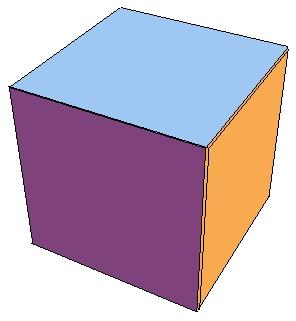cube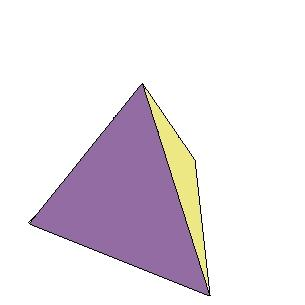tetrahedron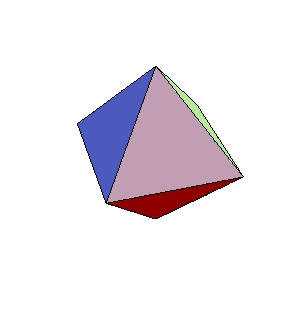octahedron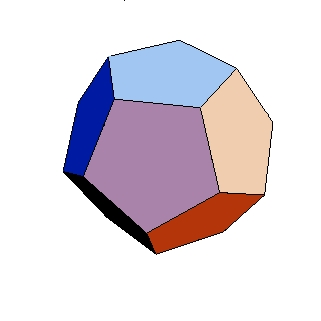dodecahedron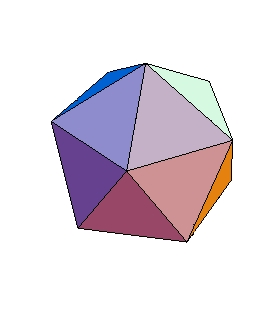icosahedron

## Exercise

Use the polydrons to make models of the 5 Platonic solids, and come up with a convincing argument that there are only 5.

## Questions

1. Why are the tetrahedron, octahedron, dodecahedron, and icosahedron called what they are called? Do you see how this naming convention is connected with the convention used to name polygons? What should the cube be called to be consistent with the names for the other 4 Platonic solids? (You occasionally see this name for the cube in the literature.)

Note: We can relax the requirements slightly and allow the faces to be different regular polygons to get semi-regular convex polyhedra. We insist that the arrangement of the polygons is the same at each vertex. For example, the truncated icosahedron is formed from regular pentagons and hexagons as on a soccer ball. At each vertex we have a pentagon, and then 2 hexagons.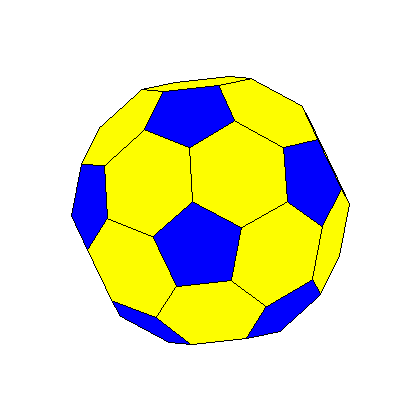truncated icosahedron

2. Do you think there are an infinite or finite number of semi-regular convex polyhedra? Play around with the polydrons to see what sort of semi-regular polyhedra you can make.

Note: There are 13 very special semi-regular polyhedra called the Archimedean solids. These solids have a lot of symmetry.

3. The soccer ball pattern above is an example of an Archimedean solid. Why do you think it is called a truncated icosahedron?

## Exercises

Perhaps you just realized that the truncated icosahedron is formed by dividing the edges of an icosahedron into thirds and then slicing the corners off of it at these points. We can perform this procedure to each of the Platonic solids and get 4 more Archimedean solids.

1. Think about what each of these polyhedron look like. What are the faces and how are they arranged at each vertex? Use the polydrons to make models of some of these polyhedra. What do you think they are called?

Pictures of the polyhedra can be found at http://home.teleport.com/~tpgettys/trplato.shtml

2. In the above procedure, we sliced the corners off of the solids a third of the way in from each vertex. What if we divide the edges in half and then truncate? How many more Archimedean solids do we get in this way?